# System Identification Using Eigensystem Realization Algorithm (ERA)

This example considers a 3-degree-of-freedom (DOF) system that is excited by a series of hammer strikes. The resulting displacements are recorded by sensors. The system is proportionally damped such that the dampling matrix is a linear combination of the mass and stiffness matrices. This example requires Signal Processing Toolbox™ for the section on modal analysis.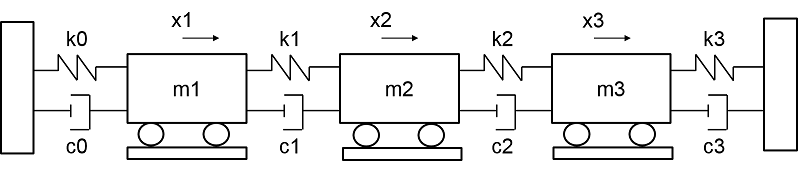### Preprocess Data

Import data for the first set of measurements. The data includes the excitation signals, reponse signals, time signals, and ground truth frequency-response functions. The response signal, denoted by Y1, gives the displacement of the first mass. The excitation signal consists of ten concatenated hammer impacts, and the response signal contains the corresponding displacement. The duration for each impact signal is 2.53 seconds. The excitation and response signals are corrupted with additive noise.

```load modaldata XhammerMISO1 YhammerMISO1 fs; rng('default'); % Add noise to excitation and response signals XhammerMISO1 = XhammerMISO1 + randn(size(YhammerMISO1))/1250; YhammerMISO1 = YhammerMISO1 + randn(size(YhammerMISO1))/1e11; % Define outputs from function t = (0:size(XhammerMISO1,1)-1)/fs'; X1 = 1e2*XhammerMISO1; Y1 = 1e2*YhammerMISO1; X0 = X1(:,1); Y0 = Y1(:,1);```

Visualize the first excitation and the response channel of the measurement.

```subplot(2,1,1) plot(t,X0) xlabel('Time (s)') ylabel('Force (N)') grid on; title('Excitation and Response for a 3DOF System') subplot(2,1,2) plot(t,Y0) xlabel('Time (s)') ylabel('Displacement (m)') grid on;```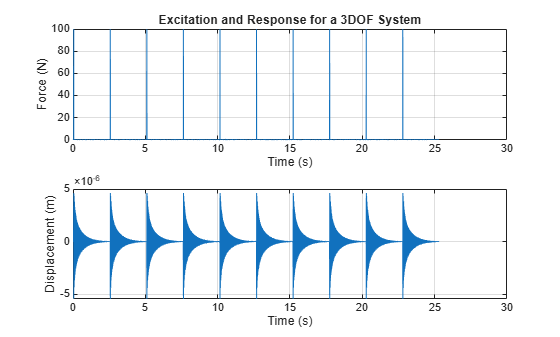### Identify System Using ERA

Create `iddata` objects for estimation and validation data.

```Ts = 1/fs; % sample time estimationData = iddata(Y0(1:1000), X0(1:1000), 1/fs); validationData = iddata(Y0(1001:2000), X0(1001:2000), 1/fs);```

Visualize the estimation data.

```figure plot(estimationData)```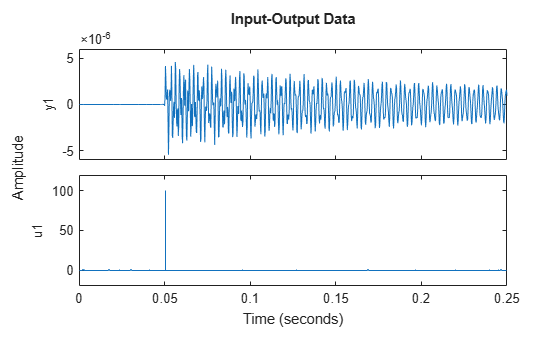The plot of the input data shows the presence of an input delay. Remove the delay from the data.

```[~,inputDelay] = max(estimationData.InputData); estimationData = estimationData(inputDelay:end);```

The `era` function requires data to be passed as a `timetable` or numeric matrix. Convert the `iddata` object into a `timetable`.

```L = length(estimationData.InputData); t = seconds(estimationData.Tstart + (0:L-1)'*Ts); y1 = estimationData.OutputData; tt = timetable(t,y1);```

Use `era` to estimate a state-space model using this estimation data.

```order = 6; sys = era(tt, order, 'Feedthrough', true)```
```sys = Discrete-time identified state-space model: x(t+Ts) = A x(t) + B u(t) + K e(t) y(t) = C x(t) + D u(t) + e(t) A = x1 x2 x3 x4 x5 x6 x1 0.8339 -0.5523 -0.001099 0.003053 -0.001497 0.0004633 x2 0.5523 0.8323 -0.00714 -0.001638 -0.002676 0.0008873 x3 -0.001099 0.00714 0.2293 0.9709 -0.0009399 -0.0006184 x4 -0.003053 -0.001638 -0.9709 0.2289 0.006094 -0.002101 x5 -0.001497 0.002676 -0.0009399 -0.006094 -0.5463 -0.8302 x6 -0.0004633 0.0008873 0.0006184 -0.002101 0.8302 -0.5465 B = u1 x1 -0.001403 x2 0.0007461 x3 -0.001515 x4 -0.0001808 x5 -0.000818 x6 -0.0002384 C = x1 x2 x3 x4 x5 x6 y1 -3.508e-07 -1.865e-07 -3.787e-07 4.52e-08 -2.045e-07 5.959e-08 D = u1 y1 1.852e-10 K = y1 x1 0 x2 0 x3 0 x4 0 x5 0 x6 0 Sample time: 0.00025 seconds Parameterization: FREE form (all coefficients in A, B, C free). Feedthrough: yes Disturbance component: none Number of free coefficients: 49 Use "idssdata", "getpvec", "getcov" for parameters and their uncertainties. Status: Estimated using the Eigensystem Realization Algorithm Model Properties ```

Compare the response of the estimated system with the validation data.

`compare(validationData,sys);`Compare the performance of the estimated model against another state-space model estimated using `ssest`.

`sys2 = ssest(estimationData, order, 'Feedthrough', true)`
```sys2 = Continuous-time identified state-space model: dx/dt = A x(t) + B u(t) + K e(t) y(t) = C x(t) + D u(t) + e(t) A = x1 x2 x3 x4 x5 x6 x1 4.99 2323 66.74 -241.3 35.31 -5.076 x2 -2341 -7.761 300.1 -11.19 11.2 25.28 x3 -49.75 -311 -5.595 -5360 30.91 15.2 x4 270.4 6.758 5333 -15.04 -1.474 -64.71 x5 -52.95 -16.58 14.17 34.15 3.021 8548 x6 -41.44 -15.95 16.8 134.7 -8674 -51.74 B = u1 x1 0.005996 x2 0.03915 x3 -0.2075 x4 -0.1881 x5 1.007 x6 -0.9297 C = x1 x2 x3 x4 x5 x6 y1 2.998e-05 -2.043e-05 1.586e-05 8.501e-06 -2.459e-06 -2.867e-06 D = u1 y1 5.33e-09 K = y1 x1 9.881e+06 x2 -8.603e+06 x3 4.435e+06 x4 2.032e+07 x5 -7.865e+07 x6 4.392e+07 Parameterization: FREE form (all coefficients in A, B, C free). Feedthrough: yes Disturbance component: estimate Number of free coefficients: 55 Use "idssdata", "getpvec", "getcov" for parameters and their uncertainties. Status: Estimated using SSEST on time domain data "estimationData". Fit to estimation data: 99.6% (prediction focus) FPE: 5.081e-17, MSE: 4.773e-17 Model Properties ```
```compare(validationData,sys,sys2); legend('Validation data','ERA','SSEST');```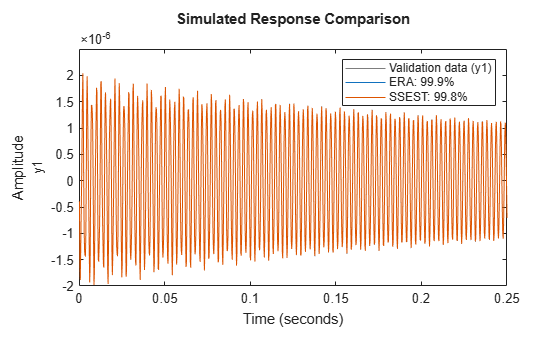The two models have a similar fit.

### Modal Analysis

Perform a modal analysis of the state-space model estimated using `era` by using the `modalfit` function from Signal Processing Toolbox. `modalfit` returns the damping ratios `zeta` and the damped natural frequencies `fd`.

```[~,f] = modalfrf(sys); [fd, zeta] = modalfit(sys,f,3);```

View `zeta`.

`zeta`
```zeta = 3×1 0.0008 0.0018 0.0028 ```

View `fd`.

`fd`
```fd = 3×1 103 × 0.3727 0.8525 1.3706 ```

Compare these results with the modes obtained from the model estimated using `ssest`.

```[frf,f] = modalfrf(sys2); [fd, zeta] = modalfit(sys2,f,3)```
```fd = 3×1 103 × 0.3727 0.8525 1.3706 ```
```zeta = 3×1 0.0008 0.0018 0.0029 ```

There is a close match between the modes obtained from `modalfit` for the second estimated model and those obtained for the model estimated using `era`.

### Reduced-order modeling comparison

Compare the performance of `era` and `ssest` when using a lower model order for system identification.

```order = 4; sys = era(tt, order, 'Feedthrough', true); sys2 = ssest(estimationData, order, 'Feedthrough', true);```

Compare the performance of both models as before.

```compare(validationData,sys,sys2); legend('Validation data','ERA','SSEST');```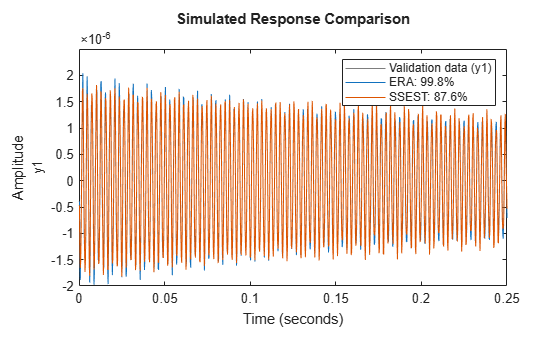For the reduced-order model, the ERA model has a significantly better fit than the SSEST model. This result demonstrates the capability of the ERA approach in obtaining state-space models from noisy impulse response data.